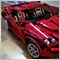# Code not working MAXDAILYLOSS341

bool Max_Loss_Daily (double PercentageLoss)
{
//datetime startTime = TimeCurrent() - Hour()*3600 - Minute()*60;
double netProfit = 0;
int total = OrdersHistoryTotal();

for(int i=total-1;i>=0;i--)
{
if(!OrderSelect(i,SELECT_BY_POS,MODE_HISTORY)) continue;
if(OrderClosePrice() == 0) continue;
//if(OrderCloseTime() < startTime) break;
netProfit += OrderProfit() + OrderSwap();
}
return(netProfit + AccountProfit() < -(AccountBalance()/100*PercentageLoss));
}

Not sure why this code isnt working but any help will be appreciated.1209

gouki1001:
Not sure why this code isnt working but any help will be appreciated.

Here's one possibility:

```bool Max_Loss_Daily (double PercentageLoss)
{
datetime startTime = iTime(Symbol(),PERIOD_D1,0);
double netProfit = 0;
int total = OrdersHistoryTotal();

for(int i=total-1;i>=0;i--)
{
if(!OrderSelect(i,SELECT_BY_POS,MODE_HISTORY)) continue;
if(OrderClosePrice() == 0) continue;
if(OrderCloseTime() < startTime) continue;
netProfit += OrderProfit() + OrderSwap();
}
double InitialBalance = AccountBalance()-netProfit;
return(netProfit + AccountProfit() < -(InitialBalance*PercentageLoss/100));
}```

Yellow: optional. Green: necessary.